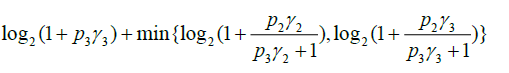# How to write the following channel capacity using CVX free error?p: corresponding scaler power, variables
gamma: scaler SNR

Convert `log2(...)` to `log(...)/log(2)`.

I think the solution at Can CVX solve this kind of function {x-log(1+0.01*x/(x+1))} can be adapted to handle each of the log terms. You’ll learn more if you do the adapting than if I do it for you.

The `min` can be left as is.

The end result should be a concave expression.

1 Like

Dear @Mark_L_Stone,
I wrote the following code for the first term in the min{} function according to your guidelines, however, {real affine}*{convex} error was shown.

log( (1+p2 * inv_pos(p3)) - (p2 * inv_pos(p3)) * inv_pos(1+p3*gamma2) )

The error includes {p2*inv_pos(p3)}.

That is not my guidance. Look again at the link, and work out the correct algebraic rearrangement, which you can easily test by putting in numbers. There will be no product terms.